JMSLTM Numerical Library 7.2.0
com.imsl.stat

## Class FactorAnalysis

• All Implemented Interfaces:
Serializable, Cloneable

```public class FactorAnalysis
extends Object
implements Serializable, Cloneable```
Performs Principal Component Analysis or Factor Analysis on a covariance or correlation matrix.

Class `FactorAnalysis` computes principal components or initial factor loading estimates for a variance-covariance or correlation matrix using exploratory factor analysis models.

Models available are the principal component model for factor analysis and the common factor model with additions to the common factor model in alpha factor analysis and image analysis. Methods of estimation include principal components, principal factor, image analysis, unweighted least squares, generalized least squares, and maximum likelihood.

For the principal component model there are methods to compute the characteristic roots, characteristic vectors, standard errors for the characteristic roots, and the correlations of the principal component scores with the original variables. Principal components obtained from correlation matrices are the same as principal components obtained from standardized (to unit variance) variables.

The principal component scores are the elements of the vector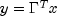where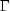is the matrix whose columns are the characteristic vectors (eigenvectors) of the sample covariance (or correlation) matrix and x is the vector of observed (or standardized) random variables. The variances of the principal component scores are the characteristic roots (eigenvalues) of the covariance (correlation) matrix.

Asymptotic variances for the characteristic roots were first obtained by Girshick (1939) and are given more recently by Kendall, Stuart, and Ord (1983, page 331). These variances are computed either for variance-covariance matrices or for correlation matrices.

The correlations of the principal components with the observed (or standardized) variables are the same as the unrotated factor loadings obtained for the principal components model for factor analysis when a correlation matrix is input.

In the factor analysis model used for factor extraction, the basic model is given as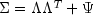where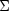is the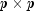population covariance matrix.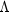is the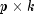matrix of factor loadings relating the factors f to the observed variables x, and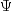is thematrix of covariances of the unique errors e. Here, p represents the number of variables and k is the number of factors. The relationship between the factors, the unique errors, and the observed variables is given as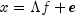where, in addition, it is assumed that the expected values of e, f, and x are zero. (The sample means can be subtracted from x if the expected value of x is not zero.) It is also assumed that each factor has unit variance, the factors are independent of each other, and that the factors and the unique errors are mutually independent. In the common factor model, the elements of the vector of unique errors e are also assumed to be independent of one another so that the matrixis diagonal. This is not the case in the principal component model in which the errors may be correlated.

Further differences between the various methods concern the criterion that is optimized and the amount of computer effort required to obtain estimates. Generally speaking, the least-squares and maximum likelihood methods, which use iterative algorithms, require the most computer time with the principal factor, principal component, and the image methods requiring much less time since the algorithms in these methods are not iterative. The algorithm in alpha factor analysis is also iterative, but the estimates in this method generally require somewhat less computer effort than the least-squares and maximum likelihood estimates. In all algorithms one eigensystem analysis is required on each iteration.

Principal Component Example, Maximum Likelihood Example, Serialized Form
• ### Nested Class Summary

Nested Classes
Modifier and Type Class and Description
`static class ` `FactorAnalysis.BadVarianceException`
`static class ` `FactorAnalysis.EigenvalueException`
Eigenvalue error.
`static class ` `FactorAnalysis.NonPositiveEigenvalueException`
Non positive eigenvalue error.
`static class ` `FactorAnalysis.NotPositiveSemiDefiniteException`
Covariance matrix not positive semi-definite.
`static class ` `FactorAnalysis.NotSemiDefiniteException`
Hessian matrix not semi-definite.
`static class ` `FactorAnalysis.RankException`
Rank of covariance matrix error.
`static class ` `FactorAnalysis.SingularException`
Covariance matrix singular error.
• ### Field Summary

Fields
Modifier and Type Field and Description
`static int` `ALPHA_FACTOR_ANALYSIS`
Indicates alpha factor analysis.
`static int` `CORRELATION_MATRIX`
Indicates correlation matrix.
`static int` `GENERALIZED_LEAST_SQUARES`
Indicates generalized least squares method.
`static int` `IMAGE_FACTOR_ANALYSIS`
Indicates image factor analysis.
`static int` `MAXIMUM_LIKELIHOOD`
Indicates maximum likelihood method.
`static int` `PRINCIPAL_COMPONENT_MODEL`
Indicates principal component model.
`static int` `PRINCIPAL_FACTOR_MODEL`
Indicates principal factor model.
`static int` `UNWEIGHTED_LEAST_SQUARES`
Indicates unweighted least squares method.
`static int` `VARIANCE_COVARIANCE_MATRIX`
Indicates variance-covariance matrix.
• ### Constructor Summary

Constructors
Constructor and Description
```FactorAnalysis(double[][] cov, int matrixType, int nf)```
Constructor for `FactorAnalysis`.
• ### Method Summary

Methods
Modifier and Type Method and Description
`double[][]` `getCorrelations()`
Returns the correlations of the principal components.
`double[][]` `getFactorLoadings()`
`double[]` `getParameterUpdates()`
`double[]` `getPercents()`
Returns the cumulative percent of the total variance explained by each principal component.
`double[]` `getStandardErrors()`
Returns the estimated asymptotic standard errors of the eigenvalues.
`double[]` `getStatistics()`
Returns statistics.
`double[]` `getValues()`
Returns the eigenvalues.
`double[]` `getVariances()`
Gets the unique variances.
`double[][]` `getVectors()`
Returns the eigenvectors.
`void` `setConvergenceCriterion1(double eps)`
Sets the convergence criterion used to terminate the iterations.
`void` `setConvergenceCriterion2(double epse)`
Sets the convergence criterion used to switch to exact second derivatives.
`void` `setDegreesOfFreedom(int ndf)`
Sets the number of degrees of freedom.
`void` `setFactorLoadingEstimationMethod(int methodType)`
`void` `setMaxIterations(int maxit)`
Sets the maximum number of iterations in the iterative procedure.
`void` `setMaxStep(int maxstp)`
Sets the maximum number of step halvings allowed during an iteration.
`void` `setVarianceEstimationMethod(int init)`
Sets the variance estimation method.
`void` `setVariances(double[] uniq)`
Sets the variances.
• ### Methods inherited from class java.lang.Object

`clone, equals, finalize, getClass, hashCode, notify, notifyAll, toString, wait, wait, wait`
• ### Field Detail

• #### ALPHA_FACTOR_ANALYSIS

`public static final int ALPHA_FACTOR_ANALYSIS`
Indicates alpha factor analysis.
Constant Field Values
• #### CORRELATION_MATRIX

`public static final int CORRELATION_MATRIX`
Indicates correlation matrix.
Constant Field Values
• #### GENERALIZED_LEAST_SQUARES

`public static final int GENERALIZED_LEAST_SQUARES`
Indicates generalized least squares method.
Constant Field Values
• #### IMAGE_FACTOR_ANALYSIS

`public static final int IMAGE_FACTOR_ANALYSIS`
Indicates image factor analysis.
Constant Field Values
• #### MAXIMUM_LIKELIHOOD

`public static final int MAXIMUM_LIKELIHOOD`
Indicates maximum likelihood method.
Constant Field Values
• #### PRINCIPAL_COMPONENT_MODEL

`public static final int PRINCIPAL_COMPONENT_MODEL`
Indicates principal component model.
Constant Field Values
• #### PRINCIPAL_FACTOR_MODEL

`public static final int PRINCIPAL_FACTOR_MODEL`
Indicates principal factor model.
Constant Field Values
• #### UNWEIGHTED_LEAST_SQUARES

`public static final int UNWEIGHTED_LEAST_SQUARES`
Indicates unweighted least squares method.
Constant Field Values
• #### VARIANCE_COVARIANCE_MATRIX

`public static final int VARIANCE_COVARIANCE_MATRIX`
Indicates variance-covariance matrix.
Constant Field Values
• ### Constructor Detail

• #### FactorAnalysis

```public FactorAnalysis(double[][] cov,
int matrixType,
int nf)```
Constructor for `FactorAnalysis`.
Parameters:
`cov` - A `double` matrix containing the covariance or correlation matrix.
`matrixType` - An `int` scalar indicating the type of matrix that is input. Uses class member `VARIANCE_COVARIANCE_MATRIX`, `CORRELATION_MATRIX` for `matrixType`.
`nf` - An `int` scalar indicating the number of factors in the model. If `nf` is not known in advance, several different values of `nf` should be used, and the most reasonable value kept in the final solution. Since, in practice, the non-iterative methods often lead to solutions which differ little from the iterative methods, it is usually suggested that a non-iterative method be used in the initial stages of the factor analysis, and that the iterative methods be used once issues such as the number of factors have been resolved.
Throws:
`IllegalArgumentException` - is thrown if `x.length`, and `x.length` are equal to 0.
• ### Method Detail

• #### getCorrelations

```public double[][] getCorrelations()
throws FactorAnalysis.RankException,
FactorAnalysis.NotSemiDefiniteException,
FactorAnalysis.NotPositiveSemiDefiniteException,
FactorAnalysis.SingularException,
FactorAnalysis.EigenvalueException,
FactorAnalysis.NonPositiveEigenvalueException```
Returns the correlations of the principal components.
Returns:
An `double` matrix containing the correlations of the principal components with the observed/standardized variables. If a covariance matrix is input to the constructor, then the correlations are with the observed variables. Otherwise, the correlations are with the standardized (to a variance of 1.0) variables. Only valid for the principal components model.
Throws:
`FactorAnalysis.RankException`
`FactorAnalysis.NotSemiDefiniteException`
`FactorAnalysis.NotPositiveSemiDefiniteException`
`FactorAnalysis.SingularException`
`FactorAnalysis.BadVarianceException`
`FactorAnalysis.EigenvalueException`
`FactorAnalysis.NonPositiveEigenvalueException`

```public double[][] getFactorLoadings()
throws FactorAnalysis.RankException,
FactorAnalysis.NotSemiDefiniteException,
FactorAnalysis.NotPositiveSemiDefiniteException,
FactorAnalysis.SingularException,
FactorAnalysis.EigenvalueException,
FactorAnalysis.NonPositiveEigenvalueException```
Returns:
A `double` matrix containing the unrotated factor loadings.
Throws:
`FactorAnalysis.RankException`
`FactorAnalysis.NotSemiDefiniteException`
`FactorAnalysis.NotPositiveSemiDefiniteException`
`FactorAnalysis.SingularException`
`FactorAnalysis.BadVarianceException`
`FactorAnalysis.EigenvalueException`
`FactorAnalysis.NonPositiveEigenvalueException`

```public double[] getParameterUpdates()
throws FactorAnalysis.RankException,
FactorAnalysis.NotSemiDefiniteException,
FactorAnalysis.NotPositiveSemiDefiniteException,
FactorAnalysis.SingularException,
FactorAnalysis.EigenvalueException,
FactorAnalysis.NonPositiveEigenvalueException```
Returns:
A `double` array containing the parameter updates when convergence was reached (or the iterations terminated). The parameter updates are only meaningful for the common factor model. The parameter updates are set to 0.0 for the principal component model.
Throws:
`FactorAnalysis.RankException`
`FactorAnalysis.NotSemiDefiniteException`
`FactorAnalysis.NotPositiveSemiDefiniteException`
`FactorAnalysis.SingularException`
`FactorAnalysis.BadVarianceException`
`FactorAnalysis.EigenvalueException`
`FactorAnalysis.NonPositiveEigenvalueException`
• #### getPercents

```public double[] getPercents()
throws FactorAnalysis.RankException,
FactorAnalysis.NotSemiDefiniteException,
FactorAnalysis.NotPositiveSemiDefiniteException,
FactorAnalysis.SingularException,
FactorAnalysis.EigenvalueException,
FactorAnalysis.NonPositiveEigenvalueException```
Returns the cumulative percent of the total variance explained by each principal component. Valid for the principal component model.
Returns:
An `double` array containing the total variance explained by each principal component.
Throws:
`FactorAnalysis.RankException`
`FactorAnalysis.NotSemiDefiniteException`
`FactorAnalysis.NotPositiveSemiDefiniteException`
`FactorAnalysis.SingularException`
`FactorAnalysis.BadVarianceException`
`FactorAnalysis.EigenvalueException`
`FactorAnalysis.NonPositiveEigenvalueException`
• #### getStandardErrors

```public double[] getStandardErrors()
throws FactorAnalysis.RankException,
FactorAnalysis.NotSemiDefiniteException,
FactorAnalysis.NotPositiveSemiDefiniteException,
FactorAnalysis.SingularException,
FactorAnalysis.EigenvalueException,
FactorAnalysis.NonPositiveEigenvalueException```
Returns the estimated asymptotic standard errors of the eigenvalues.
Returns:
An `double` array containing the estimated asymptotic standard errors of the eigenvalues.
Throws:
`FactorAnalysis.RankException`
`FactorAnalysis.NotSemiDefiniteException`
`FactorAnalysis.NotPositiveSemiDefiniteException`
`FactorAnalysis.SingularException`
`FactorAnalysis.BadVarianceException`
`FactorAnalysis.EigenvalueException`
`FactorAnalysis.NonPositiveEigenvalueException`
• #### getStatistics

```public double[] getStatistics()
throws FactorAnalysis.RankException,
FactorAnalysis.NotSemiDefiniteException,
FactorAnalysis.NotPositiveSemiDefiniteException,
FactorAnalysis.SingularException,
FactorAnalysis.EigenvalueException,
FactorAnalysis.NonPositiveEigenvalueException```
Returns statistics.
Returns:
A `double` array (Stat) containing output statistics. Stat is not defined and is set to NaN when the method used to obtain the estimates, is the principal component method, principal factor method, image factor analysis method, or alpha analysis method.  i Stat[i] 0 Value of the function minimum. 1 Tucker reliability coefficient. 2 Chi-squared test statistic for testing that the number of factors in the model are adequate for the data. 3 Degrees of freedom in chi-squared. This is computed as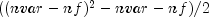where `nvar` is the number of variables and `nf` is the number of factors in the model. 4 Probability of a greater chi-squared statistic. 5 Number of iterations.
Throws:
`FactorAnalysis.RankException`
`FactorAnalysis.NotSemiDefiniteException`
`FactorAnalysis.NotPositiveSemiDefiniteException`
`FactorAnalysis.SingularException`
`FactorAnalysis.BadVarianceException`
`FactorAnalysis.EigenvalueException`
`FactorAnalysis.NonPositiveEigenvalueException`
• #### getValues

```public double[] getValues()
throws FactorAnalysis.RankException,
FactorAnalysis.NotSemiDefiniteException,
FactorAnalysis.NotPositiveSemiDefiniteException,
FactorAnalysis.SingularException,
FactorAnalysis.EigenvalueException,
FactorAnalysis.NonPositiveEigenvalueException```
Returns the eigenvalues.
Returns:
A `double` array containing the eigenvalues of the matrix from which the factors were extracted ordered from largest to smallest. If Alpha Factor analysis is used, then the first `nf` positions of the array contain the Alpha coefficients. Here, `nf` is the number of factors in the model. If the algorithm fails to converge for a particular eigenvalue, that eigenvalue is set to NaN. Note that the eigenvalues are usually not the eigenvalues of the input matrix cov. They are the eigenvalues of the input matrix cov when the `principal component` method is used.
Throws:
`FactorAnalysis.RankException`
`FactorAnalysis.NotSemiDefiniteException`
`FactorAnalysis.NotPositiveSemiDefiniteException`
`FactorAnalysis.SingularException`
`FactorAnalysis.BadVarianceException`
`FactorAnalysis.EigenvalueException`
`FactorAnalysis.NonPositiveEigenvalueException`
• #### getVariances

```public double[] getVariances()
throws FactorAnalysis.RankException,
FactorAnalysis.NotSemiDefiniteException,
FactorAnalysis.NotPositiveSemiDefiniteException,
FactorAnalysis.SingularException,
FactorAnalysis.EigenvalueException,
FactorAnalysis.NonPositiveEigenvalueException```
Gets the unique variances.
Returns:
A `double` array of length nvar containing the unique variances, where `nvar` is the number of variables.
Throws:
`FactorAnalysis.RankException`
`FactorAnalysis.NotSemiDefiniteException`
`FactorAnalysis.NotPositiveSemiDefiniteException`
`FactorAnalysis.SingularException`
`FactorAnalysis.BadVarianceException`
`FactorAnalysis.EigenvalueException`
`FactorAnalysis.NonPositiveEigenvalueException`
• #### getVectors

```public double[][] getVectors()
throws FactorAnalysis.RankException,
FactorAnalysis.NotSemiDefiniteException,
FactorAnalysis.NotPositiveSemiDefiniteException,
FactorAnalysis.SingularException,
FactorAnalysis.EigenvalueException,
FactorAnalysis.NonPositiveEigenvalueException```
Returns the eigenvectors.
Returns:
A `double` matrix containing the eigenvectors of the matrix from which the factors were extracted. The j-th column of the eigenvector matrix corresponds to the j-th eigenvalue. The eigenvectors are normalized to each have Euclidean length equal to one. Also, the sign of each vector is set so that the largest component in magnitude (the first of the largest if there are ties) is made positive. Note that the eigenvectors are usually not the eigenvectors of the input matrix cov. They are the eigenvectors of the input matrix cov when the `principal component` method is used.
Throws:
`FactorAnalysis.RankException`
`FactorAnalysis.NotSemiDefiniteException`
`FactorAnalysis.NotPositiveSemiDefiniteException`
`FactorAnalysis.SingularException`
`FactorAnalysis.BadVarianceException`
`FactorAnalysis.EigenvalueException`
`FactorAnalysis.NonPositiveEigenvalueException`
• #### setConvergenceCriterion1

`public void setConvergenceCriterion1(double eps)`
Sets the convergence criterion used to terminate the iterations.
Parameters:
`eps` - A `double` used to terminate the iterations. For the least squares and and maximum likelihood methods convergence is assumed when the relative change in the criterion is less than `eps`. For alpha factor analysis, convergence is assumed when the maximum change (relative to the variance) of a uniqueness is less than `eps`. `eps` is not referenced for the other estimation methods. If this member function is not called, `eps` is set to 0.0001.
• #### setConvergenceCriterion2

`public void setConvergenceCriterion2(double epse)`
Sets the convergence criterion used to switch to exact second derivatives.
Parameters:
`epse` - A `double` used to switch to exact second derivatives. When the largest relative change in the unique standard deviation vector is less than `epse` exact second derivative vectors are used. If this member function is not called, `epse` is set to 0.1. Not referenced for principal component, principal factor, image factor, or alpha factor methods.
• #### setDegreesOfFreedom

`public void setDegreesOfFreedom(int ndf)`
Sets the number of degrees of freedom.
Parameters:
`ndf` - An `int` value specifying the number of degrees of freedom in the input matrix. If this member function is not called 100 degrees of freedom are assumed.

`public void setFactorLoadingEstimationMethod(int methodType)`
Parameters:
`methodType` - An `int` scalar indicating the method to be applied for obtaining the factor loadings. Use class member `PRINCIPAL_COMPONENT_MODEL`, `PRINCIPAL_FACTOR_MODEL`, `UNWEIGHTED_LEAST_SQUARES`, `GENERALIZED_LEAST_SQUARES`, `MAXIMUM_LIKELIHOOD`, `IMAGE_FACTOR_ANALYSIS`, or `ALPHA_FACTOR_ANALYSIS` for `methodType`. If this member function is not called, the `PRINCIPAL_COMPONENT_MODEL` is used.

For the principal component and principal factor methods, the factor loading estimates are computed as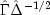whereand the diagonal matrix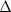are the eigenvalues and eigenvectors of a matrix. In the principal component model, the eigensystem analysis is performed on the sample covariance (correlation) matrix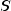while in the principal factor model the matrix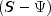is used. If the unique error variancesare not known in the principal factor model, then they are estimated. This is achieved by calling the member function `setVarianceEstimationMethod` and setting `init` to 0. If the principal component model is used, the error variances are set to 0.0 automatically.

The basic idea in the principal component method is to find factors that maximize the variance in the original data that is explained by the factors. Because this method allows the unique errors to be correlated, some factor analysts insist that the principal component method is not a factor analytic method. Usually however, the estimates obtained via the principal component model and other models in factor analysis will be quite similar.

It should be noted that both the principal component and the principal factor methods give different results when the correlation matrix is used in place of the covariance matrix. Indeed, any rescaling of the sample covariance matrix can lead to different estimates with either of these methods. A further difficulty with the principal factor method is the problem of estimating the unique error variances. Theoretically, these must be known in advance and passed in through member function `setVariances`. In practice, the estimates of these parameters produced by calling the member function `setVarianceEstimationMethod` and setting `init` to 0 are often used. In either case, the resulting adjusted covariance (correlation) matrix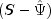may not yield the `nf` positive eigenvalues required for `nf` factors to be obtained. If this occurs, the user must either lower the number of factors to be estimated or give new unique error variance values.

For the least-squares and maximum likelihood methods an iterative algorithm is used to obtain the estimates (see Joreskog 1977). As with the principal factor model, the user may either input the initial unique error variances or allow the algorithm to compute initial estimates. Unlike the principal factor method, the code then optimizes the criterion function with respect to bothand. (In the principal factor method,is assumed to be known. Given, estimates formay be obtained.)

The major differences between the estimation methods described in this member function are in the criterion function that is optimized. Letdenote the sample covariance (correlation) matrix, and letdenote the covariance matrix that is to be estimated by the factor model. In the unweighted least-squares method, also called the iterated principal factor method or the minres method (see Harman 1976, page 177), the function minimized is the sum of the squared differences betweenand. This is written as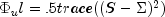.

Generalized least-squares and maximum likelihood estimates are asymptotically equivalent methods. Maximum likelihood estimates maximize the (normal theory) likelihood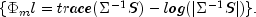while generalized least squares optimizes the function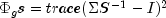.

In all three methods, a two-stage optimization procedure is used. This proceeds by first solving the likelihood equations forin terms ofand substituting the solution into the likelihood. This gives a criterion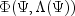, which is optimized with respect to. In the second stage, the estimates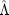are obtained from the estimates for.

The generalized least-squares and the maximum likelihood methods allow for the computation of a statistic for testing that `nf` common factors are adequate to fit the model. This is a chi-squared test that all remaining parameters associated with additional factors are zero. If the probability of a larger chi-squared is small (see `stat` under `getStatistics`) so that the null hypothesis is rejected, then additional factors are needed (although these factors may not be of any practical importance). Failure to reject does not legitimize the model. The statistic `stat` is a likelihood ratio statistic in maximum likelihood estimates. As such, it asymptotically follows a chi-squared distribution with degrees of freedom given in `stat`.

The Tucker and Lewis (1973) reliability coefficient,, is returned in `stat` when the maximum likelihood or generalized least-squares methods are used. This coefficient is an estimate of the ratio of explained to the total variation in the data. It is computed as follows: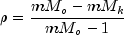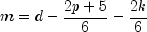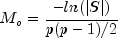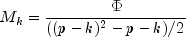where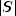is the determinant of `cov`, p is the number of variables, k is the number of factors,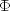is the optimized criterion, and d is the number of degrees of freedom.

The term "image analysis" is used here to denote the noniterative image method of Kaiser (1963). It is not the image factor analysis discussed by Harman (1976, page 226). The image method (as well as the alpha factor analysis method) begins with the notion that only a finite number from an infinite number of possible variables have been measured. The image factor pattern is calculated under the assumption that the ratio of the number of factors to the number of observed variables is near zero so that a very good estimate for the unique error variances (for standardized variables) is given as one minus the squared multiple correlation of the variable under consideration with all variables in the covariance matrix.

First, the matrix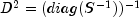is computed where the operator "diag" results in a matrix consisting of the diagonal elements of its argument, andis the sample covariance (correlation) matrix. Then, the eigenvaluesand eigenvectorsof the matrix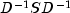are computed. Finally, the unrotated image factor pattern matrix is computed as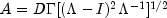.

The alpha factor analysis method of Kaiser and Caffrey (1965) finds factor-loading estimates to maximize the correlation between the factors and the complete universe of variables of interest. The basic idea in this method is as follows: only a finite number of variables out of a much larger set of possible variables is observed. The population factors are linearly related to this larger set while the observed factors are linearly related to the observed variables. Let f denote the factors obtainable from a finite set of observed random variables, and letdenote the factors obtainable from the universe of observable variables. Then, the alpha method attempts to find factor-loading estimates so as to maximize the correlation between f and. In order to obtain these estimates, the iterative algorithm of Kaiser and Caffrey (1965) is used.

• #### setMaxIterations

`public void setMaxIterations(int maxit)`
Sets the maximum number of iterations in the iterative procedure.
Parameters:
`maxit` - An `int` used as the maximum number of iterations allowed during the iterative portion of the algorithm. If this member function is not called, `maxit` is set to 60. Not referenced for factor loading methods principal component, principal factor, or image factor methods.
• #### setMaxStep

`public void setMaxStep(int maxstp)`
Sets the maximum number of step halvings allowed during an iteration.
Parameters:
`maxstp` - An `int` used as the maximum number of step halvings allowed during an iteration. If this member function is not called, `maxstp` is set to 8. Not referenced for principal component, principal factor, image factor, or alpha factor methods.
• #### setVarianceEstimationMethod

`public void setVarianceEstimationMethod(int init)`
Sets the variance estimation method.
Parameters:
`init` - An `int` used to designate the method to be applied for obtaining the initial estimates of the unique variances. If this member function is not called, `init` is set to 1.  init Method 0 Initial estimates are taken as the constant 1-nf/(2*nvar) divided by the diagonal elements of the inverse of input matrix `cov`, where `nvar` is the number of variables. 1 Initial estimates are input by the user in vector `uniq (setVariances)`.
Note that when the factor loading estimation method is PRINCIPAL_COMPONENT_MODEL, the initial estimates in `uniq` are reset to 0.0.
• #### setVariances

`public void setVariances(double[] uniq)`
Sets the variances.
Parameters:
`uniq` - A `double` array of length nvar containing the unique variances, where `nvar` is the number of variables. If this member function is not called, the elements of uniq are set to 0.0. If the iterative methods fail for the unique variances used, new initial estimates should be tried. These may be obtained by use of another factoring method (use the final estimates from the new method as initial estimates in the old method). Another alternative is to call member function `setVarianceEstimationMethod` and set the input argument to 0. This will cause the initial unique variances to be estimated by the code.
JMSLTM Numerical Library 7.2.0